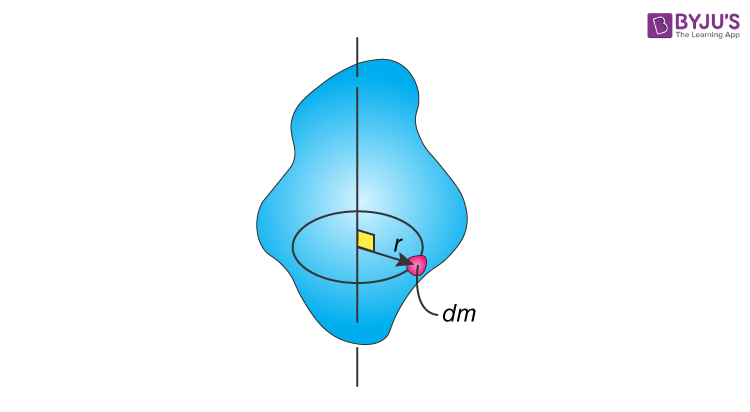Checkout JEE MAINS 2022 Question Paper Analysis : Checkout JEE MAINS 2022 Question Paper Analysis :

# Mass Moment Of Inertia

Mass moment of inertia also known as rotational inertia is a quantity that is used in measuring a body’s resistance to a change in its rotation direction or the angular momentum. It basically characterizes the acceleration undergone by an object or solid when torque is applied. The mass moment of inertia is usually denoted by the letter I.

If we talk about the relationship, when there is a given moment or torque applied on a body, if the body’s mass moment of inertia is larger its angular acceleration will be lower. Meanwhile, I also affect how a body stores kinetic energy in rotation.

## Key Points To Remember

• Mass Moment of Inertia depends on the body’s mass and the location of the mass.
• If the distance of the mass is farther from the rotational axis, the mass moment of inertia will be relatively larger.

## Mathematical Equations To Calculate Mass Moment Of InertiaIf we take the point mass the MOI can be represented as;

I = m r2

If we closely analyze this equation, the mass moment of inertia will have units of mass times length squared. However, the mass moment of inertia should not be confused with the area moment of inertia. On the other hand, mass moments of inertia are usually a part of the equations of motion and it relays information on how much inertia there is to rotate the particle around a given axis.

More significantly, point mass is taken as the basis for all other moments of inertia. The moment of inertia of other objects can be calculated from the sum of the moments.

I = ∑i mi ri2 = m1 r12 + m2 r22 + ….. + mn rn2

If we want to calculate the mass moment of inertia of rigid bodies, we take the sum of particles where each body will have a certain mass (dm). While the calculation might be complex, we use integration to sum the moment of inertia of each dm and obtain the desired result. The formula is also expressed as an integral. It is given as;

IG = ∫ r2 dm

Mass moment of inertia is also used in different rigid body rotation problems. These include;

 ( ΣMG = IGα ) F = ma analysis moment equation ( T = ½ IGω2 ) Rotational kinetic energy ( HG = IGω ) Angular momentum

Mass moment of inertia units are kg.m2 and lb.in2.

## Significance

To sum up, the mass moment of inertia clearly indicates what degree of resistance is offered by a body to rotational acceleration about an axis. If a body has a large mass moment of inertia it can be said or deduced that it offers high resistance to angular acceleration.

## Parallel Axis Theorem## Frequently Asked Questions on Mass Moment of Inertia

### What is mass moment of inertia?

The rotational analog of mass is the mass moment of inertia. The mass moment of inertia should be used in all rotational equations of angular momentum, angular kinetic energy, force, and so on.

### What is the unit of mass moment of inertia?

The unit of mass moment of inertia is kgm2.

### What are the three types of moment of inertia?

Mass Moment of Inertia
Area Moment of Inertia
Polar Moment of Inertia

Test your knowledge on Mass Moment Of Inertia